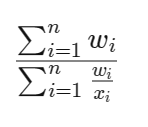###### Tax Troubles? We've Got You Covered!
Get Expert Consultation Now!
•2500 Cr. Saved Already!
•Trusted by 1 Million+
•10+ Yr. of Industry Experience# Harmonic Mean

## What is harmonic mean?

The harmonic mean is a type of average that is especially useful when working with ratios or rates. It is calculated by dividing the number of values ​​in the data series by the sum of the reciprocals of each value in the series. Compared to other types of averages, such as arithmetic and geometric means, harmonic averages give equal weight to each data point and are suitable when values ​​are in a ratio or rate relationship.

In finance, the harmonic mean finds application in determining the average for financial multiples, such as the price-to-earnings (P/E) ratio. When averaging financial multiples, it is not advisable to use the arithmetic mean because it can be biased towards larger values, potentially distorting the overall average. The harmonic mean provides a more balanced approach by giving equal weight to each value, which is desirable in cases where the values represent different securities in a portfolio, for example. Thus, the harmonic mean helps in calculating the ratio of a portfolio that consists of multiple securities by providing a fair representation of the average.

## The Basics of Harmonic Mean

The harmonic mean is a useful tool for determining the multiplicative or divisor relationship between fractions, eliminating the need to consider common denominators. It is commonly employed when averaging rates, such as calculating the average travel speed based on the duration of multiple trips.

In finance, the weighted harmonic mean is particularly relevant for averaging multiples like the price-to-earnings (P/E) ratio. This approach assigns equal weight to each data point, ensuring a fair representation.

On the other hand, if a weighted arithmetic mean were used to average these ratios, higher data points would receive greater importance due to the absence of price normalization, while the earnings would be equalized.

The harmonic mean can be seen as a special case of the weighted harmonic mean, where the weights assigned to each data point are equal to 1. In the weighted harmonic mean, the values x1, x2, and x3 are multiplied by their corresponding weights w1, w2, w3, and then summed. This sum is divided by the sum of the weights w1, w2, and w3. Mathematically, it can be represented asThis calculation yields the weighted harmonic mean of the values x1, x2, x3, with the weights w1, w2, and w3.